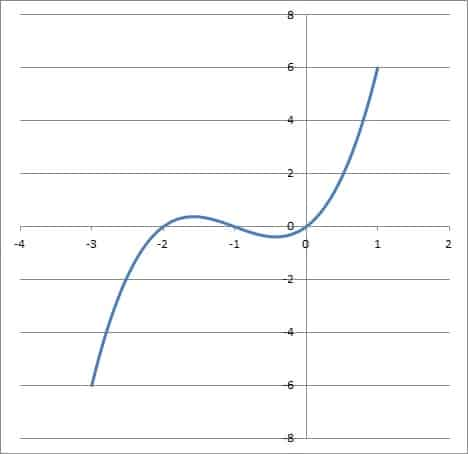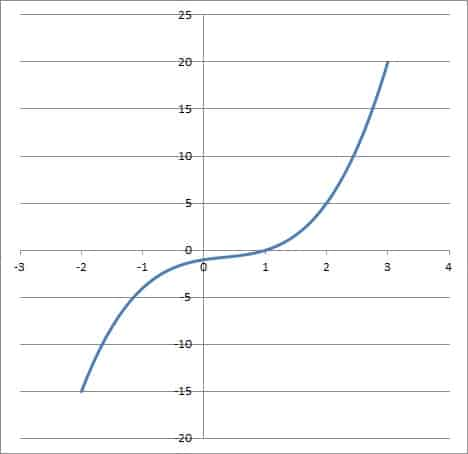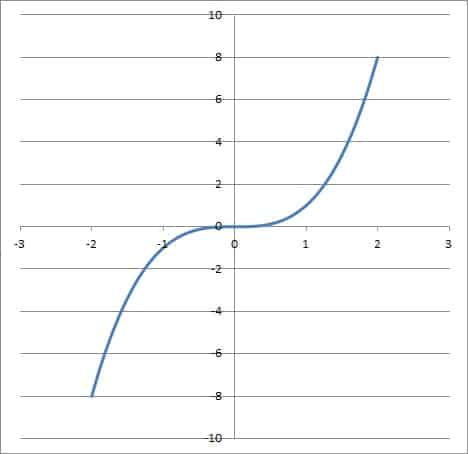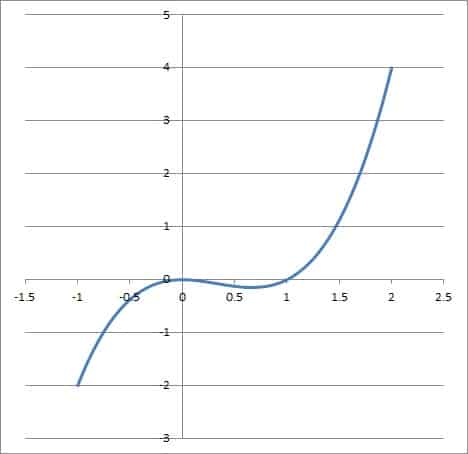# Cubic Functions (10 Common Questions Answered)

Cubic functions are useful for modeling the volume of objects (such as spheres or boxes).  However, there is a lot to know about these third degree polynomials, their roots, and their graphs.

So, what do you need to know about cubic functions?  A cubic function has the form f(x) = ax3 + bx2 + cx + d, where a, b, c, and d are real with a not zero. Some cubic functions are one to one, and some have odd symmetry, but no cubic function has even symmetry. A cubic function has 3 roots, and it has either zero or two complex or imaginary roots.

Of course, a cubic function may have up to two local extrema (maximums or minimums), and it always has an inflection point (since the second derivative is linear).

In this article, we’ll take a closer look at cubic functions and answer some common questions about them.  We’ll also look at some examples, including graphs of various cubic functions.

Let’s get started.

## What Is A Cubic Function?

A cubic function is one that has the standard form

• f(x) = ax3 + bx2 + cx + d

where a, b, c, and d are real, with a not equal to zero.

A cubic function is also called a third degree polynomial, or a polynomial function of degree 3.  This means that x3 is the highest power of x that has a nonzero coefficient.

This also means that a cubic polynomial can be described as an odd-degree polynomial.  This means that it will always have at least one real root, and it will always have an odd number of real roots (and an even number of complex roots, which come in conjugate pairs).

The domain of a cubic function is the set of all real numbers.  The range of a cubic function is also the set of all real numbers.

If we set a cubic function equal to zero, we get a cubic equation:

• f(x) = 0

or

• ax3 + bx2 + cx + d = 0

This equation has 3 solutions.  Some of the solutions may be repeated, and some of them may be complex or imaginary numbers.

Here are some examples of cubic functions and their graphs.

### Example 1: A Cubic Function & Its Graph

Consider the cubic function

• f(x) = x3 + 3x2 + 2x

Note that a = 1, b = 3, c = 2, and d = 0.

We can set this function equal to zero and factor it to find the roots, which will help us to graph it:

• f(x) = 0
• x3 + 3x2 + 2x = 0
• x(x2 + 3x + 2) = 0
• x(x + 1)(x + 2) = 0

So the roots are x = 0, x = -1, and x = -2.  This means that the graph touches the x-axis in three places: at x = 0, -1, and -2.

We will use these values to help us graph the function:The cubic function f(x) = x3 + 3x2 + 2x has zeros at x = 0, x = -1, and x = -2.

Note that the function approaches negative infinity as x becomes more negative.  The function approaches positive infinity as x becomes more positive.

### Example 2: A Cubic Function & Its Graph

Consider the cubic function

• f(x) = x3 – x2 + x – 1

Note that a = 1, b = -1, c = 1, and d = -1.

We can set this function equal to zero and factor it to find the roots, which will help us to graph it:

• f(x) = 0
• x3 – x2 + x – 1 = 0
• (x3 – x2) + (x – 1) = 0
• x2(x – 1) + (x – 1) = 0
• (x2 + 1)(x – 1) = 0
• (x + i)(x – i)(x – 1) =

So the roots are x = 1, x = i, and x = -i (where I = √-1).  So, this cubic only has one real zero, meaning that the graph only touches the x-axis once (at x = 1).

The graph of the function is pictured below:The cubic function f(x) = x3 – x2 + x – 1 has zeros at x = 1, x = i, and x = -i. The imaginary zeros do not appear on the graph.

Note that the function approaches negative infinity as x becomes more negative.  The function approaches positive infinity as x becomes more positive.

## What Is A Cubic Function Used For In Real Life?

For one thing, a cubic function can be used to model the volume of objects, including boxes and spheres.

### Example 1: Volume Of A Box With A Square Base

Let’s say we have a box with a square base.  The length of a side of the square base is x.

We want the box to be twice as tall as it is wide.  Then the height of the box is 2x.

The volume of a box is:

• V = (length)*(width)*(height)

In this case, we have a length and width of x (the square base) and a height of 2x (twice the width).  So, our volume becomes:

• V = (x)*(x)*(2x)
• V = 2x3

So, we can choose a value of x and find the corresponding volume of the box we described.  For example, if the side length is x = 3 feet, then the volume is V = 2(3)3 = 54 cubic feet.

We can also choose a volume for this box and work backwards to find the dimensions.  For example, let’s say we want this type of box to have a volume of 128 cubic feet.

We need to solve the volume equation for 128:

• V = 2x3
• 128 = 2x3
• 64 = x3
• 4 = x

So, the length and width of the box is 4 (a base with area 16 square feet).  The height is 2x = 8 feet.

### Example 2: Volume Of A Sphere

If a sphere has radius x, then its volume is given by the equation

• V = (4πx3) / 3

This is a cubic function, since x3 is the highest power of x with a nonzero coefficient in a polynomial.

## How Can You Tell If A Graph Is Cubic?

There are some signs to look for to tell if a graph is cubic:

• The first derivative, f’(x), will be quadratic.  So, it will be zero for either 0, 1, or 2 real values.  This means that the cubic f(x) can have zero, one, or at most two local extrema (maximums or minimums).
• The second derivative, f’’(x), will be linear.  So, the cubic f(x) will have a single inflection point where it changes concavity.  https://jdmeducational.com/when-is-a-function-concave-or-convex-4-key-ideas/
• The shape of the graph: see the examples below for a > 0 and a < 0.

### How Do You Shift A Cubic Function?

To shift a cubic function up or down by k units (a vertical shift), simply add or subtract k from the function.

For example, if f(x) = x3 is our original cubic function, then:

• g(x) = f(x) + k = x3 + k is the graph of f(x) shifted up by k units.
• h(x) = f(x) – k = x3 – k is the graph of f(x) shifted down by k units.

To shift a cubic function left or right by h units (a horizontal shift), simply subtract or add h from every x in the function.

For example, if f(x) = x3 is our original cubic function, then:

• g(x) = f(x + h) = (x + h)3 is the graph of f(x) shifted left by h units.
• h(x) = f(x – h) = (x – h)3 is the graph of f(x) shifted right by h units.

If you want to shift the graph both horizontally and vertically, then combine both types of shifts.  For example, the function j(x) = f(x – h) + k is the graph of f(x) shifted h units right and k units up.

## How Do You Tell If A Function Is Cubic From A Table?

To tell if a function is cubic from a table, extend the table by adding 5 columns: first differences of y, first differences of x, the quotient of the first y differences with the first x differences, and then two more columns: the 2nd and 3rd differences of the quotient.

Look at the last 3 columns of the table.  If all of the values in this last column are the same, but the other two columns have varying values, then the table is from a cubic function.

### Example 1: A Cubic Function From A Table

Consider the following table of values:

We will extend the table as follows:

Now we look at the last 3 columns.  Note that all of the values in the last column are the same, while the other two columns have differing values.

This means that the third derivative is constant, which tells us that the table is from a cubic function: f(x) = x3 + 2.

### Example 2: A Non-Cubic Function From A Table

Consider the following table of values:

We will extend the table as follows:

Now we look at the last 3 columns.  Note that all of the values in the last column are the same, as are the values in the 2nd to last column.

This means that the second and third derivatives are constant, which tells us that the table is from a quadratic function: f(x) = 2x2 – 1.

## Is A Cubic Function One To One?

Not all cubic functions are one to one, but some of them are.

### Example 1: A Cubic Function That Is One To One

Consider the cubic function

• f(x) = x3

This function is one to one, as we can see from the graph below:The cubic function f(x) = x3 is one to one, since it passes the horizontal line test.

We can also see this algebraically by using the horizontal line test.  Any horizontal line has the equation y = k.

If k is a real number, then it has a real cube root, r = k1/3.  We can then set the cubic equal to k and solve to find how many points of intersection there are:

• f(x) = k
• x3 = r3  [r = k1/3, or r3= k]
• x3 – r3 = 0
• (x – r)(x2 + rx + r2) = 0  [factor as a difference of cubes]

This implies x = r or x2 + rx + r2 = 0.  The second possibility is a quadratic equation with a = 1, b = r, and c = r2.

In this case, the discriminant (the expression under the radical in the quadratic formula) is:

• Discriminant = b2 – 4ac
• Discriminant = r2 – 4(1)(r2)
• Discriminant = r2 – 4r2
• Discriminant = –3r2

Since the discriminant is negative, we know that the quadratic has two complex conjugate roots and no real roots.  Thus, there is only one real solution to the cubic, and only one point where the horizontal line y = k intersects the cubic f(x) = x3.

### Example 2: A Cubic Function That Is Not One To One

Consider the cubic function

• f(x) = x3 + 3x2 + 2x

This cubic function is not one to one, since it fails the horizontal line test.  This is easy to see if we use the horizontal line y = 0.

Solving for the intersection of the cubic and line, we get:

• f(x) = 0
• x3 + 3x2 + 2x = 0
• x(x2 + 3x + 2) = 0
• x(x + 1)(x + 2) = 0

This means that x = 0, x = -1, and x = -2 are all solutions.  So, the horizontal line y = 0 intersects the cubic f(x) = x3 + 3x2 + 2x at three points: (0, 0), (-1, 0), and (-2, 0).The cubic function f(x) = x3 + 3x2 + 2x is not one to one, since the horizontal line y = 0 intersects the graph three times.

The cubic fails the horizontal line test, so it is not one to one.

## Can A Cubic Function Have Two Zeros?

A cubic function can have two zeros if it has two real roots, one of which is repeated twice.  For example, consider the cubic function

• f(x) = x3 – x2

We can factor this easily as:

• f(x) = x2(x – 1)

This means that the cubic is equal to zero when x = 0 or x = 1.  Thus, there are two real roots.

However, x = 0 is repeated (double) root.The cubic function f(x) = x3 – x2 has two zeros: x = 0 (a double root) and x = 1.

Similarly, a cubic function have one zero if it is a triple root (repeated three times).  For example, f(x) = x3 has one zero (x = 0), and it is a triple root.

## Can A Cubic Function Have Three Imaginary Roots? (No Real Roots)

A cubic function cannot have three imaginary roots. This means that a cubic function must have at least one real root.

It can have either two imaginary roots (a complex conjugate pair) or no complex roots.

According to the Fundamental Theorem of Algebra, a polynomial of degree n with real coefficients has n complex roots (counting repeated roots).  Applying this to a cubic with real coefficients (n = 3), we can see that such a function has 3 roots (possibly repeated roots).

A cubic function can have imaginary roots in some cases.  A cubic function will always have either 0 or 2 imaginary roots, which must be complex conjugates of one another (according to the Complex Conjugate Root Theorem).

For example, if x = 2i is a root of a cubic f(x), then x = -2i (the complex conjugate of 2i) is also a root of f(x).  The third root of this cubic would be real.

The tables below summarizes the possibilities for real and complex or imaginary roots of cubic functions.

## Does A Cubic Function Have Asymptotes?

A cubic function does not have any asymptotes.

A cubic function has no vertical asymptotes, since there is no possibility of a zero denominator in a cubic polynomial.

A cubic function has no horizontal asymptotes, since the range of a cubic polynomial is all real numbers.

## Does A Cubic Function Have Symmetry? (Even/Odd)

Some cubic functions have symmetry, but not all.

There is no even cubic function, since there is guaranteed to be an odd power of x (specifically, x3).  So, a cubic function cannot be even.

A cubic function is odd if it has no quadratic term and no constant term (that is, if the coefficient of x2 is zero and the constant term is also zero).

### Example 1: An Odd Cubic Function

Consider the cubic function

• f(x) = x3 + x

We can show that f(-x) = -f(x):

• f(-x) = (-x)3 + (-x)
• f(-x) = -x3 – x  [a negative raised to an odd power is negative]
• f(-x) = -(x3 + x)  [factor out -1]
• f(-x) = -f(x)  [f(x) = x3 + x]

So, f(x) = x3 + x is an odd cubic function.

### Example 2: A Cubic Function That Is Not Odd

Consider the cubic function

• f(x) = x3 + 1

We can show that f(x) is not odd

• f(-x) = (-x)3 + 1
• f(-x) = -x3 + 1  [a negative raised to an odd power is negative]
• f(-x) = -(x3 – 1)  [factor out -1]

Since f(-x) = -(x3 – 1) and -f(x) = -(x3 + 1), we can see that f(-x) is not equal to –f(x).  So, f(x) = x3 + 1 is not odd.